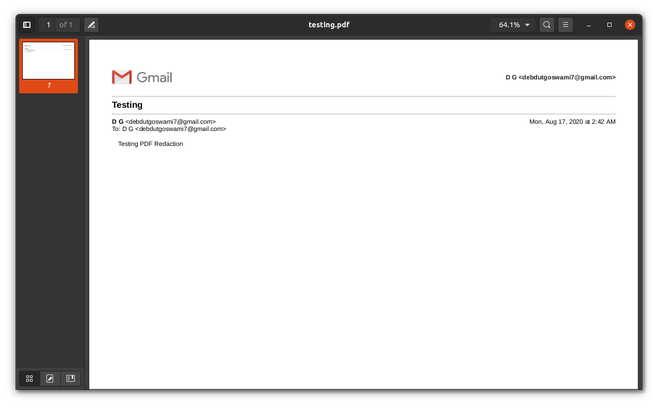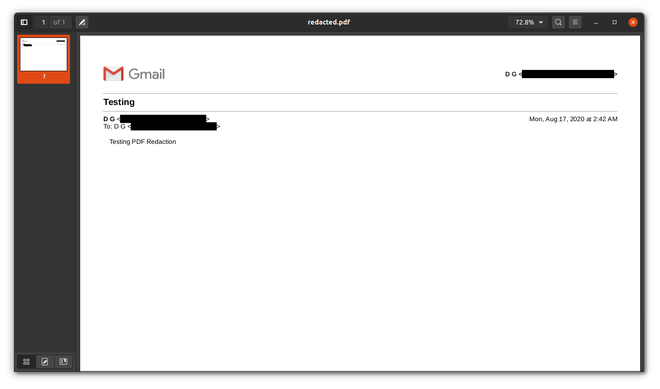# PDF Redaction using Python

• Last Updated : 22 Jun, 2021

So, let’s just start with what exactly does Redaction mean. So, Redaction is a form of editing in which multiple sources of texts are combined and altered slightly to make a single document. In simple words, whenever you see any part in any document which is blackened out to hide some information, it is known as Redaction. To perform the same task on a PDF is known as PDF Redaction.

If anyone has worked with any kind of data extraction on PDF, then they know how painful it can be to handle PDFs. Consider a scenario where you want to share a PDF with someone but there are certain parts in a PDF that you don’t want to get leaked. So, what you can do is, you can redact the texts. It is pretty easy to redact texts using something like Adobe Acrobat, but what if you want this to be an automated process. Suppose, you are working in a company that shares its user’s purchases on its site with the Income Tax Department but due to strict privacy policies, the safety of users’ Personal Identifiable Information (PII) they want to remove those from the transaction receipts. If the user base is large then it can not be done manually, so you need some kind of automation to do so. This is where Python comes in. There is this amazing library called PyMuPDF, which is a library for pdf handling and performing various operations on them. So, let’s just check out how we are going to do so.

First, you need to have Python3 installed and also PyMuPDF installed. To install PyMuPDF, simply open up your terminal and type the following in it

`pip3 install PyMuPDF`

For this demonstration, we will be only redacting Email IDs from a PDF. You can apply the same logic to any other PII

Approach:

1. Read the PDF file
2. Iterate line by line through the pdf and look for each occurrence of any email id. Email IDs have a pattern, so we will be using Regex to identify an email
3. Once we encounter an email, we add it to a list and then return the list at the end of the last line
4. Now, we need to simply search for the occurrence of the fetched email ids in the pdf. PyMuPDF makes it very easy to find any text in a PDF. It returns four coordinates of a rectangle inside which the text will be present.
5. Once we have all the text boxes, we can simply iterate over those boxes and Redact each box from the PDF

Below is the implementation of the above approach and I have added inline comments for a better understanding of the code.

PDF file used:Before

## Python3

 `# imports``import` `fitz``import` `re` `class` `Redactor:``  ` `    ``# static methods work independent of class object``    ``@staticmethod``    ``def` `get_sensitive_data(lines):``      ` `        ``""" Function to get all the lines """``        ` `        ``# email regex``        ``EMAIL_REG ``=` `r``"([\w\.\d]+\@[\w\d]+\.[\w\d]+)"``        ``for` `line ``in` `lines:``          ` `            ``# matching the regex to each line``            ``if` `re.search(EMAIL_REG, line, re.IGNORECASE):``                ``search ``=` `re.search(EMAIL_REG, line, re.IGNORECASE)``                ` `                ``# yields creates a generator``                ``# generator is used to return``                ``# values in between function iterations``                ``yield` `search.group(``1``)` `    ``# constructor``    ``def` `__init__(``self``, path):``        ``self``.path ``=` `path` `    ``def` `redaction(``self``):``      ` `        ``""" main redactor code """``        ` `        ``# opening the pdf``        ``doc ``=` `fitz.``open``(``self``.path)``        ` `        ``# iterating through pages``        ``for` `page ``in` `doc:``          ` `            ``# _wrapContents is needed for fixing``            ``# alignment issues with rect boxes in some``            ``# cases where there is alignment issue``            ``page._wrapContents()``            ` `            ``# getting the rect boxes which consists the matching email regex``            ``sensitive ``=` `self``.get_sensitive_data(page.getText(``"text"``)``                                                ``.split(``'\n'``))``            ``for` `data ``in` `sensitive:``                ``areas ``=` `page.searchFor(data)``                ` `                ``# drawing outline over sensitive datas``                ``[page.addRedactAnnot(area, fill ``=` `(``0``, ``0``, ``0``)) ``for` `area ``in` `areas]``                ` `            ``# applying the redaction``            ``page.apply_redactions()``            ` `        ``# saving it to a new pdf``        ``doc.save(``'redacted.pdf'``)``        ``print``(``"Successfully redacted"``)` `# driver code for testing``if` `__name__ ``=``=` `"__main__"``:``  ` `    ``# replace it with name of the pdf file``    ``path ``=` `'testing.pdf'``    ``redactor ``=` `Redactor(path)``    ``redactor.redaction()`

Output:After

My Personal Notes arrow_drop_up